# How to determine power gain and voltage gain in RF systems

Other Parts Discussed in Post: LMH6521, LMH6515

I’m hearing more and more customers ask ”how does gain through a signal chain change with different load impedances?"  And, "when does voltage gain and power gain coincide when measured in dB?” I wanted to share the answers with the Analog Wire audience in case any of you have the same quesitons. So, here we go…..

In a single-ended signal path with 50 Ohms termination, the gain calculations are very easy because the voltage gain (20* log (Vout/Vin)) is equal to the power gain (10* log (Pout/Pin)).  Things become a bit more complicated though when the impedance of the load, or source, changes.   For example, in many radio receiver channels the 50 Ohm single-ended signal is converted to a 200 Ohm differential signal before it it digitized with a high performance ADC, such as the ADC16DV160.

In addition, there are two main types of amplifiers, voltage output amplifiers like the LMH6521, and current output amplifiers like the LMH6515.   The calculations below show how these two different kinds of amplifiers react to different load conditions.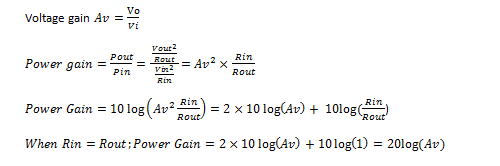Voltage output amplifiers are the most common amplifiers in non-RF systems and have been immortalized by the classic operational amplifier (op amp). Note that both current feedback and voltage feedback op amps have a voltage output architecture.   With recent developments in bipolar transistor technology, op amps and their derivatives are useful up to 2 GHz or higher. As a result,  they are finding their way into RF and IF signal paths.  Because an op amp has infinite input impedance and zero output impedance,  the power gain of an op amp is not normally specified, rather the gain is given as a voltage gain (Av).  A common gain setting is 6dB, where the output voltage is 2x the input voltage.  Note that this gain does not specify an input load condition or an output load condition.  Because a voltage alone is not sufficient to calculate power, power gain can not be calculated using only a voltage gain.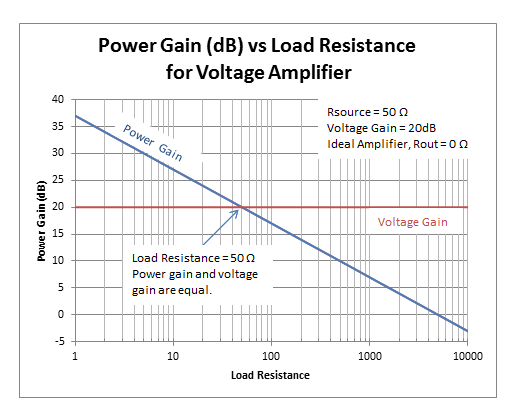Figure 1 ideal voltage amplifier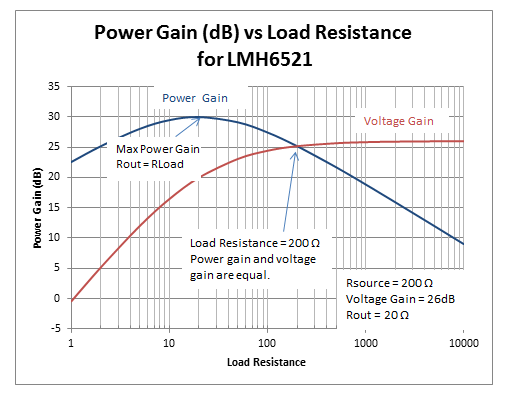Figure 2 example voltage amplifier: LMH6521

Current output  amplifiers are another common type of RF amplifier beacause a given input signal a given output current is produced.  There are two common configurations in one configuration Iout = (in * Gain) in the other configuration Iout = (Vin * gain).  The latter is more common and in that case the gain is called transconductance (gm). In transconductance  amplifier calculations, both the voltage gain and power gain are dependent on load conditions. (Example Amplifier LMH6515 Rin = 200 Ω, Rout = 200 Ω or 400 Ω, Maximum  gain = 0.1 A/V)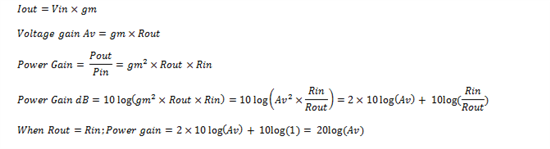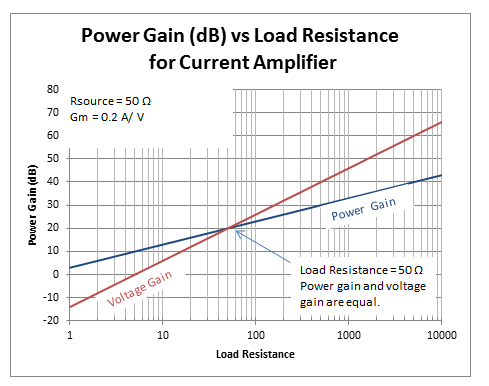Figure 3 ideal current amplifier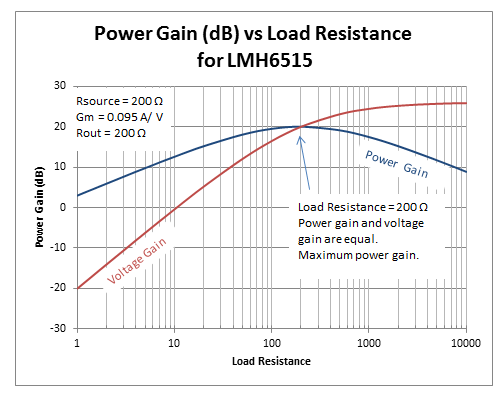Figure 4 example current amplifier: LMH6515

For both amplifier topologies, voltage gain in dB and power gain in dB are only equal when the input and output impedances are the same.   With current amplifiers, however, both voltage gain and power gain will change with load conditions, while with voltage amplifiers only power gain changes with load.

As an exercise for the reader, prove that a back terminated load cuts both voltage gain and power gain by 6dB and let me know how it goes!

Parents
• Hello Stevan,

For the purposes of this blog I did not consider Source resistance.  The graphs that show real devices have the device output resistance included in the calculation.  With a given IC such as the LMH6517 the output resistance is fixed and close to zero and so it does not factor into the calculation very much.  On a part like the LMH6521 the output resistance is fixed, but is not negligible, so it must be considered.  For the LMH6515 the output resistance can be chosen from two different values, so it is good to know the tradeoffs of the selections.

I did not include source resistance calculations because many times test equipment is already calibrated to take into account a matched load.  For example, an RF signal generator wit a 50 Ohm output will deliver the indicated power into a 50 Ohm load.  It is correct that if you connect a different load to the signal generator you will get a different power, so this is an important consideration.

When we make measurements most of the time we take great care to provide matched conditions for our test equipment.   For a 200 Ohm DVGA we would use a 1:4 balun on the input to convert the 50 Ohm signal generator signal to 200 Ohms for the DVGA.  If magnetic components do not work we will use a resistive pad to provide the impedance matching.

Comment
• Hello Stevan,

For the purposes of this blog I did not consider Source resistance.  The graphs that show real devices have the device output resistance included in the calculation.  With a given IC such as the LMH6517 the output resistance is fixed and close to zero and so it does not factor into the calculation very much.  On a part like the LMH6521 the output resistance is fixed, but is not negligible, so it must be considered.  For the LMH6515 the output resistance can be chosen from two different values, so it is good to know the tradeoffs of the selections.

I did not include source resistance calculations because many times test equipment is already calibrated to take into account a matched load.  For example, an RF signal generator wit a 50 Ohm output will deliver the indicated power into a 50 Ohm load.  It is correct that if you connect a different load to the signal generator you will get a different power, so this is an important consideration.

When we make measurements most of the time we take great care to provide matched conditions for our test equipment.   For a 200 Ohm DVGA we would use a 1:4 balun on the input to convert the 50 Ohm signal generator signal to 200 Ohms for the DVGA.  If magnetic components do not work we will use a resistive pad to provide the impedance matching.

Children
No Data